1 Tutorials that teach Representing How Data is Normally Distributed

# Representing How Data is Normally Distributed

Author: Sophia Tutorial
##### Description:

Identify features of a normal distribution curve.

(more)

Sophia’s self-paced online courses are a great way to save time and money as you earn credits eligible for transfer to many different colleges and universities.*

No credit card required

37 Sophia partners guarantee credit transfer.

299 Institutions have accepted or given pre-approval for credit transfer.

* The American Council on Education's College Credit Recommendation Service (ACE Credit®) has evaluated and recommended college credit for 32 of Sophia’s online courses. Many different colleges and universities consider ACE CREDIT recommendations in determining the applicability to their course and degree programs.

Tutorial
what's covered
This lesson discusses representing how data is normally distributed. The key objective for this lesson is to understand a normal distribution, and be able to identify the mean, median, and mode when looking at a graph of a bell curve. This lesson will cover:
1. Bell-Shaped Curves
2. Normal Distribution
3. Measures of Center

## 1. Bell-Shaped Curves

Now that you have some basic understanding of data and how it is measured, it is important to know how the data is distributed. Simply knowing the mean, median, or mode is generally not sufficient to explain much about the data. Knowing just how much the data strays away from those measures is important as well.

Knowing how data is distributed allows us to make predictions about what to expect and what not to expect for a given data set. Graphically, smooth curves, called density curves, can be used to show the distribution of variables. Look at the graph shown here. Notice how the curve is smooth and shaped like a bell.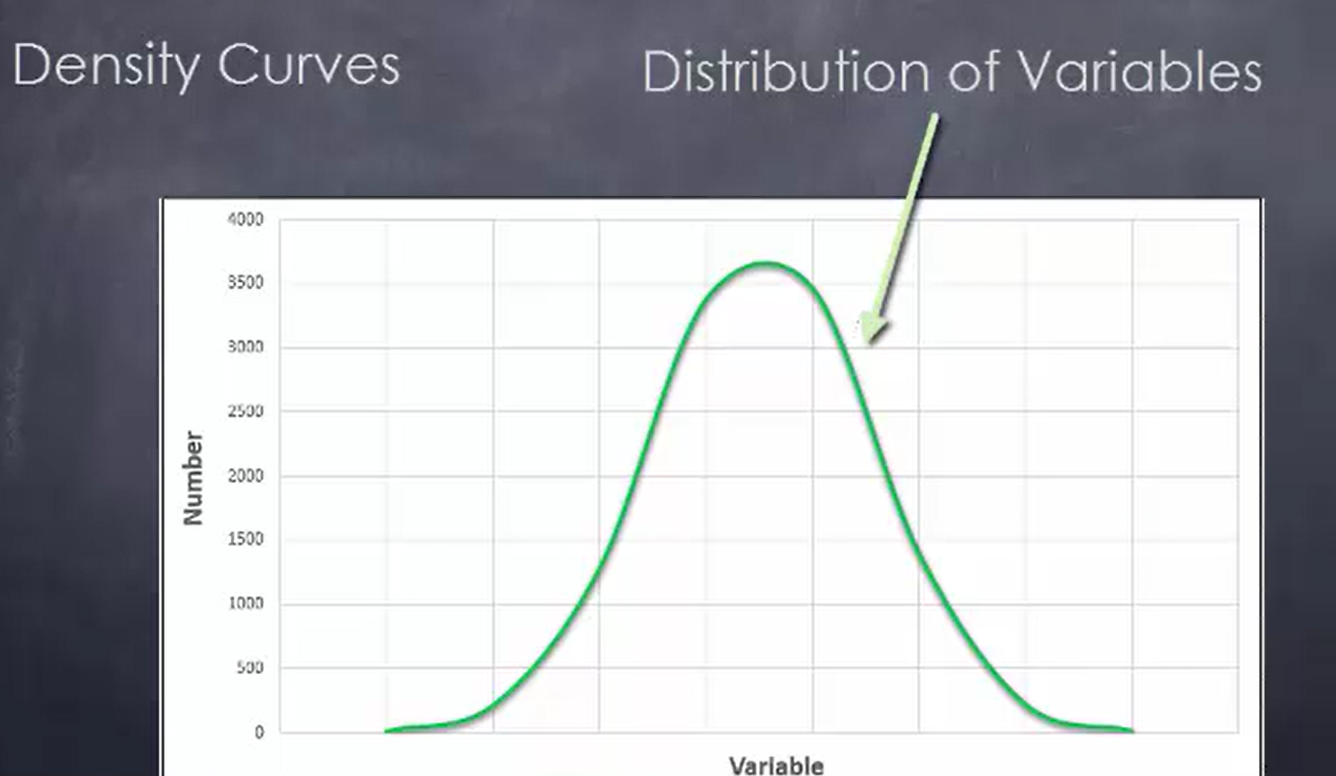What’s useful about a density curve is that it shows how often a given value was recorded. What happens to create this curve is that a large number of observations are taken and how often they happen is plotted out. Eventually, you’ll get a shape that resembles a bell.

## 2. Normal Distribution

Take a look at this curve that represents IQ scores, which are a measure of intelligence. The horizontal axis represents the actual score. Notice that the bell-shaped curve is drawn above the horizontal axis. It’s centered at 100, which is the average IQ.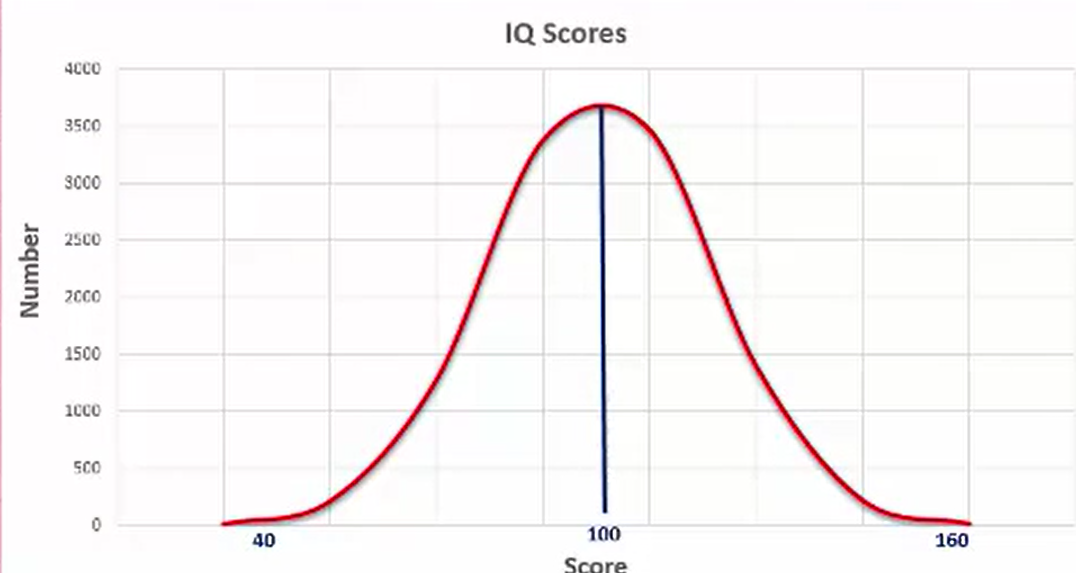The range of this bell-shaped curve goes from, at the low end, 40 to the high end at 160. This type of curve is called a normal distribution curve, and they occur quite often in many different situations.

## 3. Measures of Center

This next graph represents time spent on social media per day by teenagers. It is another bell-shaped curve. You can see that the average, or the mean, is centered at 500 minutes per day. The range is 200 to 800 minutes per day. The number of hypothetical teenagers—10,000—is shown on the left-hand side.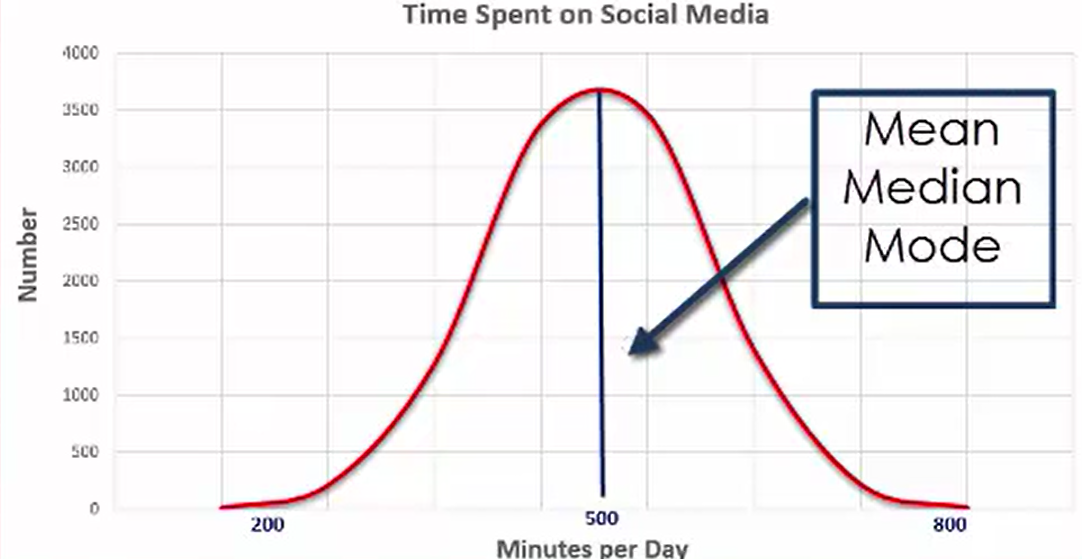Notice how the large number of observations are concentrated more toward the center. When you’re looking at normal distributions, they all have density curves that are symmetric and bell shaped. The mean, median, and mode of the normal distribution are all the same and equal to the center value of the density curve, which in this case is 500.

Check out a distribution curve for the height of college basketball players indicated in this graph: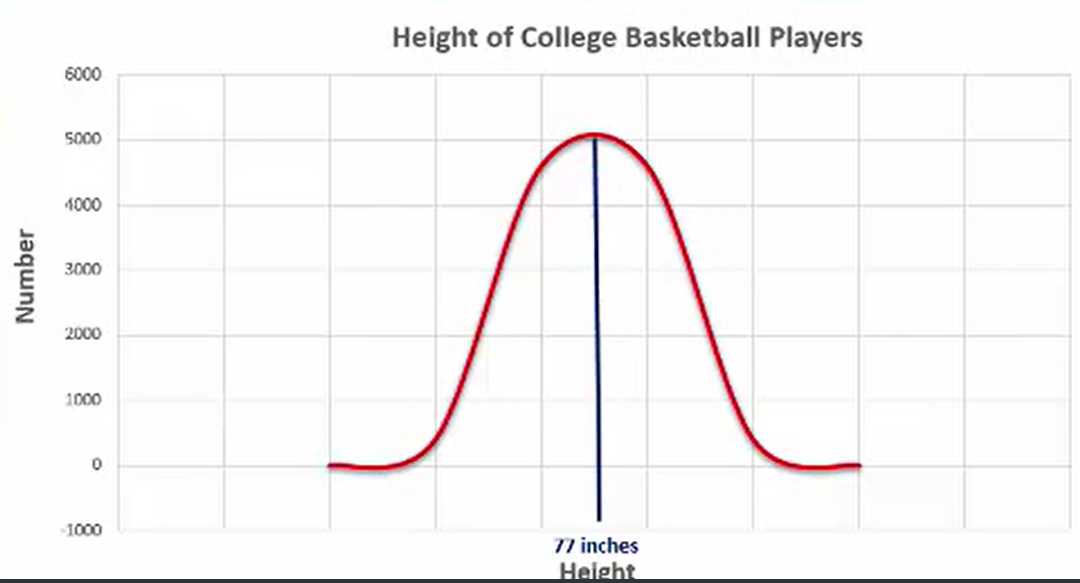try it
Determine the mean, median and mode for this curve.

Notice that the mean, median, and mode are all the same: 77 inches.

The distribution is relatively narrow. Most of the players are clustered right around that midpoint. There’s not a whole lot of variation from that actual mean of 77 inches.

Now here’s a distribution of SAT scores. The average in this case is 1,497, which was actually the average SAT score in the year 2012. Notice how this curve deviates a little further from the mean, median, and mode. The curve looks a little flatter in this instance, with a wider distribution with regard to how the observations actually fall.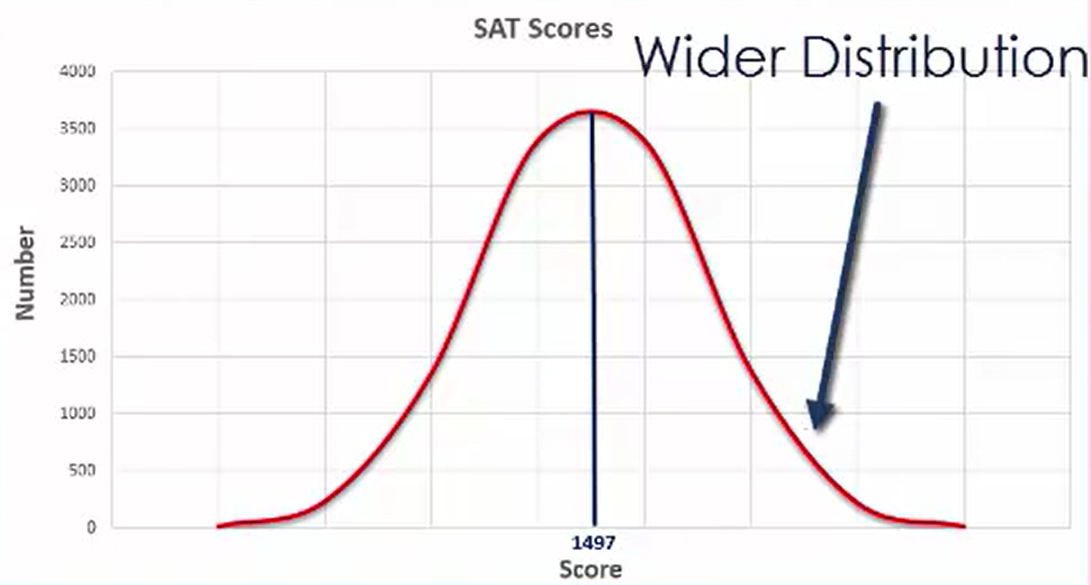summary
You were introduced to bell-shaped curves in this lesson. You also came to understand what a normal distribution is. By looking at this type of graph, you learned how to easily identify the measures of center, mean, median, and mode. It’s going to be that center point at the very top of the bell curve that has the most observations.

Source: This work is adapted from Sophia author Dan Laub.

Rating